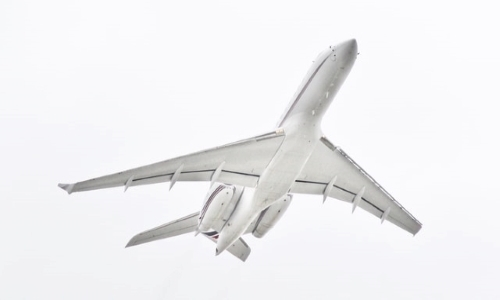# PID Controller Design for Aircraft Pitch LoopAn aircraft controller incorporates several control loops to manage the speed, altitude, and navigation of an airplane. The aircraft pitch control is a vital inner loop for stability augmentation and holds autopilot controllers for the longitudinal dynamics. In this tutorial, we will show how to design a PID tuner for the aircraft pitch control loop

## Aircraft Pitch System Model

The aircraft pitch system variables are defined in Figure 1. The pitch angle $$\theta$$ is the angle between the horizon direction and the x-axis of the aircraft's body. The angle of attack $$\alpha$$ is the angle between the aircraft's longitudinal axis and the wind direction $$v$$. The pitch angle is manipulated through the deflection in the elevator $$\delta_e$$.

Through system identification, we can get the following numerical differential equations for aircraft pitch dynamics:

$$\dot\alpha=-0.298\alpha+54.3q+0.223\delta_e$$

$$\dot q=-0.0141\alpha-0.399q+{0.0199\delta}_e$$

$$\dot\theta=54.3q$$

We can convert the above differential equations into a state-space form:

$$\left[\begin{matrix}\dot\alpha\\\dot{q}\\\dot\theta\\\end{matrix}\right]=\left[\begin{matrix}-0.298&54.3&0\\-0.0141&-0.399&0\\0&54.3&0\\\end{matrix}\right]\left[\begin{matrix}\alpha\\q\\\theta\\\end{matrix}\right]+\left[\begin{matrix}0.223\\0.0199\\0\\\end{matrix}\right]\delta_e$$

$$y=\left[\begin{matrix}0&0&1\\\end{matrix}\right]\left[\begin{matrix}\alpha\\q\\\theta\\\end{matrix}\right]$$

Before defining the state space model into Collimator, we will add some useful libraries:

import numpy as np
import matplotlib.pyplot as plt
import control as ctrl
import collimator as C



The state-space matrices are defined in the Collimator notebook as follows.

A = np.matrix([[-0.298, 54.3, 0],
[-0.0141, -0.399, 0],
[0, 54.3, 0]])
B = np.matrix([0.223, 0.0199, 0]).T
C = np.matrix([0, 0, 1])
D = 
pitch_sys = ctrl.ss(A,B,C,D)
print(pitch_sys)


<Linear IOSystem>: sys
Inputs (1): ['u']
Outputs (1): ['y]
States (3): ['x', 'x', 'x']

A = [[-2.98e-01 5.43e+01
(-1.41e-02 -3.99e-01 [ 0.08e+00 5.43e+01
0.002+00] 0.00+00) 0.002+00]]

B = [[8.223 ]
[0.0199) [e. ]]

C = [[0. 0. 1.]] D = [(0.]]



## Analysis and PID Controller Design

A look at the poles and zeros of the aircraft pitch system gives us the following results.

print(ctrl.zero(pitch_sys))
print(ctrl.pole(pitch_sys))


(-0.13999497+0.j]
[ 0. +0.j-2.3485+0.87354436j -0.3485-0.87354436j]



We can see that the system has an integrator. This will make the system marginally stable, though it can get unstable for some bounded signals. The system also has a zero which will make the time-domain characteristics laws unreliable for design considerations.

Now, we will take a look at the open-loop and closed-loop step responses for the pitch control system. For the closed-loop system, we will apply a proportional controller with $$k_p=1$$ for comparative reasons.

plt.figure(figsize=(12,8))
plt.grid(which='both')
T, yout = ctrl.step_response(0.2*pitch_sys,T=np.arange(0, 20, 0.01))
plt.plot(T, yout,label='Open Loop')
pitch_sys_closed = ctrl.feedback(pitch_sys,1)
T, yout = ctrl.step_response(0.2*pitch_sys_closed,T=np.arange(0, 60, 0.01))
plt.plot(T, yout,label='Closed Loop')
plt.legend()



We can see that the open-loop step response is growing in an unbounded direction. This is due to the shortcomings of the integrator dynamics as we explained earlier, and the closed-loop step response though stable is unsatisfying:

ctrl.step_info(0.2*pitch_sys_closed)


{'RiseTime': 18.699415428900895,
'SettlingTime': 39.395855806519364,
'SettlingMin': 0.18021917292358314,
'SettlingMax': 0.19999999999999998,
'Overshoot': 0,
'Undershoot': 0,
'Peak': 0.19991079488520766,
'PeakTime': 87.50600229835176,



Due to the integrator dynamics, the system exhibits no steady-state error, but it’s very slow in terms of rise time and settling time. In order to ensure satisfactory standards are met, The flight quality performance measures must meet the following design requirements:

• Overshoot $$\le 15%$$.
• Rise time $$\le 0.5$$ seconds.
• Settling time $$\le 4$$ seconds.
• Steady-state error $$\le 1%$$.

A PID controller is added in the loop as depicted in Figure 2.

In the next cell, we will create an interactive PID tuner so that we can see how the step responses change as we update the controller gain. We will start designing the controller by evaluating some empirical PID gains. For this purpose, we will use ipywidgets library within our Collimator notebook. First, we will define a function that computes the step response and time-domain characteristics for the output step response.

def interactive_tuning(kp = 1, ki=0, kd=0):
s = ctrl.tf('s')
pid = kp+ki/s+kd*10000*s/(s+10000)
pitch_y = ctrl.feedback(pid*pitch_sys,1)
print(ctrl.step_info(0.2*pitch_y))
plt.figure(figsize=(12,8))
T, y = ctrl.step_response(0.2*pitch_y,T=np.arange(0, 50, 0.1))
plt.grid(which='both')
plt.plot(T, y)
plt.ylabel('Pitch Angle')
plt.xlabel('Time')



We now define the interactive variables for the tuning function. We will assume a range of $$\left[0,10\right]$$ for the PID gains.

from ipywidgets import interact, fixed
interact(interactive_tuning,kp = (0,10,0.01),ki = (0,10,0.01),kd = (0,10,0.01))



We can increase the $$P$$ gain until we introduce some oscillation to increase the system speed. Then, we will increase the integrator gain to make the controller more aggressive. Finally, we will introduced the derivate gain to maintain system speed while damping the aggressive oscillation.

Now, we can see that the PID gains meet the design requirements.